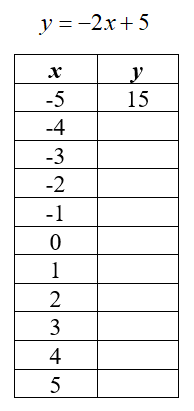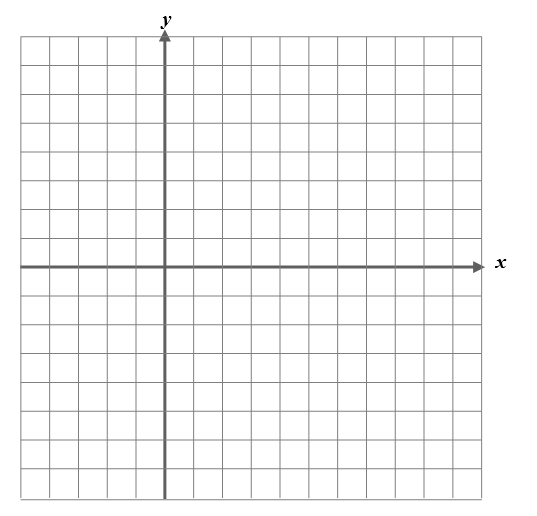# Try This! (Tables and Graphs)

The linear situation you explored in the last section could be represented by the equation $$y=-2x+5$$, where $$x$$ represents the number of minutes walking and $$y$$ represents your current elevation.  According to this equation, after 15 minutes of walking, your elevation is $$-25$$, which means that you are 25 feet below the elevation of the center of campus.

The linear equations that you graphed in Lesson 2 modeled a specific situation.  Although the context of a problem helps you understand what the answer means, it is not necessary to know the context of the problem in order to graph from an equation. Next, you will create a table and graph and interpret a linear equation without context.

##### Part 1: Graph
1. Complete the data table below by substituting the $$x$$-values from the table into the formula to find the $$y$$-values.
2. Use a coordinate plane, like the one below, to graph and connect the ordered pairs from the table in the graph provided.
TableExample: Use $$x=-5$$

$y=-2(-5)+5\\\\y=10+5\\\\y=15$

Graph

$y=-2x+5$##### Part 2: Interpret
1. Find the slope of this line.  What method did you use?
2. Explain where you find the slope in the equation $$y=-2x+5$$.
3. What is the $$y$$-intercept of this line?  (Be sure to name the ordered pair.)
4. Explain where you find the $$y$$-intercept in the equation $$y=-2x+5$$.

Note: The slope-intercept form of a line is given by the equation $$y=mx+b$$, where $$m$$ represents the slope and $$b$$ represents the $$y$$ intercept.

Watch the video located at the link below to learn more about graphing lines from equations in the form $$y=mx+b$$.

Graphing Linear Equations | MyOwlsLearning